Courses

# Test: Binomial Numbers (Competition Level)- 2

## 25 Questions MCQ Test Mathematics For JEE | Test: Binomial Numbers (Competition Level)- 2

Description
This mock test of Test: Binomial Numbers (Competition Level)- 2 for JEE helps you for every JEE entrance exam. This contains 25 Multiple Choice Questions for JEE Test: Binomial Numbers (Competition Level)- 2 (mcq) to study with solutions a complete question bank. The solved questions answers in this Test: Binomial Numbers (Competition Level)- 2 quiz give you a good mix of easy questions and tough questions. JEE students definitely take this Test: Binomial Numbers (Competition Level)- 2 exercise for a better result in the exam. You can find other Test: Binomial Numbers (Competition Level)- 2 extra questions, long questions & short questions for JEE on EduRev as well by searching above.
QUESTION: 1

### Let n be a positive integer. Then of the following, the greatest term is

Solution:

i)(1+1/4n)4n
= (1+1/4)4
= (5/4)4 = 625/256

ii)(1+1/3n)3n
= (1+1/3)3
= (4/3)3 = 64/27

iii)(1+1/2n)2n
=(1+1/2)2 = (3/2)2
= 6/4 = 3/2

iv)(1+1/n)n
=(1+1)1 = 2

QUESTION: 2

Solution:
QUESTION: 3

### The last two digits of the number 3400 are

Solution:
QUESTION: 4

The sum of the co-efficients of all the even powers of x in the expansion of (2x2 – 3x + 1)11 is

Solution:
QUESTION: 5

The coefficient of xr(0 < r < n – 1) in the expression (x + 2)n-1 + (x + 2)n-2 . (x + 1) + (x + 2)n-3. (x + 1)2 + .............+ (x + 1)n- is

Solution:
QUESTION: 6

The co-efficient of x401 in the expansion of (1 + x + x2 + ........+x9)-1, (|x| < 1) is

Solution:
*Multiple options can be correct
QUESTION: 7

Let (1 + x2)2 (1 + x)n = A0 + A1x + A2x2 + ........... If A0, A1, A2 are in A.P. then the value of n is

Solution:
*Multiple options can be correct
QUESTION: 8

The number 101100 – 1 is divisible by

Solution:
*Multiple options can be correct
QUESTION: 9

If the 6th term in the expansion of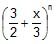when x = 3 is numerically greatest then the possible integral value(s) of n can be

Solution:
*Multiple options can be correct
QUESTION: 10

If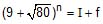where I, n are integers and 0 < f < 1, then

Solution:
QUESTION: 11

Positive integers can be represented as

Solution:

*Multiple options can be correct
QUESTION: 12

In the expansion of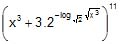Solution:
*Multiple options can be correct
QUESTION: 13

The co-efficient of the middle term in the expansion of (1 + x)2n is

Solution:
*Multiple options can be correct
QUESTION: 14

The value of nC0 . nCn + nC1 . nCn – 1 + ... + nCn . nC0 is

Solution:
*Multiple options can be correct
QUESTION: 15

The value of r for which 30C20C30Cr – 1 . 20C+...+ 30C20C r is maximum, is

Solution:
*Multiple options can be correct
QUESTION: 16

The value of r(0 < r < 30) for which 20C10C20Cr – 1 . 10C+...+ 20C10Cis minimum, is

Solution:
*Multiple options can be correct
QUESTION: 17

The value of r(0 < r < 30) for which 20C10C20Cr – 1 . 10C+...+ 20C10Cis minimum, is

Solution:
QUESTION: 18

Column-I Column-II

(A) If l be the number of terms in the expansion of (P) O + T = 3

(1 + 5x + 10x2 + 10x2 + 5x4 + x5)20 and if unit's

place and ten's place digits in 3are O and T, then

(B) If l be the number of terms in the expansion (Q) O + T = 7

of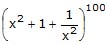and if unit's place and (R) O + T = 9

ten's place digits in 7are O and T, then

(C) If l be the number of terms in the

expansion of (1 + x)101 (1 + x2 _ x)100 (S) T _ O = 7

and if unit's place and ten's place

digits in 9l are O and T, then (T) O _ T = 7

subjective type

Solution:
QUESTION: 19

Find the coefficients

(i) x7 in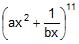(ii) x _7 in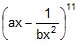(iii) Find the relation between a and b, so that these coefficients are equal.

Solution:

Correct Answer :- ab = 1

Explanation : rth term  in the expansion of (a + b)ⁿ is given by nCr(a)r(b)n-r

To find the coefficient of x⁷ in (ax² + 1/bx)¹¹

rth term is given by 11Cr(ax²)r (1/bx)11-r

= 11Cr ar (1/b)11-r x(r-22+2r)

=11Cr ar (1/b)11-r x3r-22

To find the coefficient of x⁷, 3r - 11 should be 7

=> 3r - 11 = 7

=> 3r = 18

=> r = 6

Thus the coefficient of x⁷ is 11C6 a⁶b⁻⁵

To find the coefficient of x⁻⁷ in (ax - 1/bx²)¹¹

rth term is given by 11Cr = (ax)r(-1/bx²)11-r

= 11Cr ar (-1/b)11-r x(r-22+2r)

=11Cr ar (1/b)11-r x3r-22

To find the coefficient of x⁻⁷, 3r - 22 should be -7

=> 3r - 22 = -7

=> 3r = 15

=> r = 5

Thus the coefficient of x⁷ is 11C(a)⁵(-1/b)⁶ = 11C6 a⁵b⁻⁶

Given that these coefficients are equal

=>11C6 a⁶b⁻⁵ = 11C6 a⁵b⁻⁶

⇒ a = 1/b

⇒ ab = 1

*Answer can only contain numeric values
QUESTION: 20

If the coefficients of (2r + 4)th , (r – 2)th terms in the expansion of (1 + x)18 are equal, find r.

Solution:

In the expansion of (1+x)18

coefficient of T2r+4​ = 18C2r+3

coefficient of Tr−2 ​= 18Cr−3

Given,

18C2r+3 ​= 18Cr−3​

⇒ 2r−3 = r+3   or   2r+3+r−3 = 18

⇒ r = −6              or         3r = 18 ⇒ r = 6

but r cannot be negative

∴ r = 6

QUESTION: 21

If the coefficients of the rth, (r + 1)th and (r + 2)th terms in the expansion of (1 + x)14 are in A.P., find r.

Solution: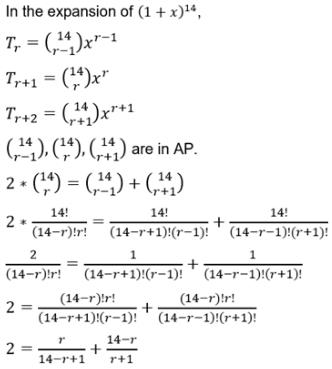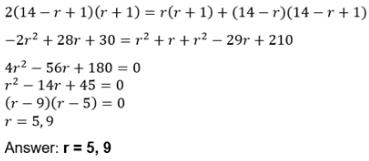QUESTION: 22

Find the term independent of x in the expansion of

(a)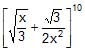(b)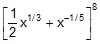Solution:

QUESTION: 23

Given that (1 + x + x2)= a0 + a1x + a2x2 + ...... +a2nx2n, find the values of

(i) a0 + a1 + a2 + ..... + a2n ;

(ii) a0 _ a1 + a2 _ a3 ....... +a2n ;

(iii) a02 _ a12 + a22 _ a32 + ... + a2n2

Solution:
QUESTION: 24

In a Binomial Distribution, if ‘n’ is the number of trials and ‘p’ is the probability of success, then the mean value is given by

Solution:
QUESTION: 25

Prove that :

n _ 1Cr n _ 2Cr n _ 3Cr + ............ + rCr nCr + 1.

Solution: